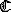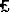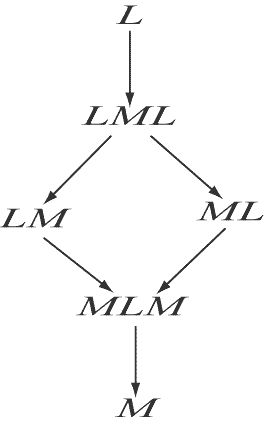## THE SYSTEMS OF COMPLETE MODALIZATION -- S4°, S4, AND S5

The system S4°

WE now turn to other systems based on S1°. As before, we add to S1° an axiom, in this case:

M9.MMpMp

This formula plus S1° yields S4° (Sobocinski 1962). As a theorem of S4° we clearly have

 11.1LpLLp M9, duality

With 4.1 as a thesis, we shall be able to prove in S4° theorems of form LLa. As we established in our work on the system , S1° plus any theorem beginning with two L's contains the system , in which the rule to infer La from any thesis a holds. So S4° clearly containsand the last mentioned rule, which may be called RL as in Chapter 7.

Since it containsS1°, S4° has as a thesis formula 5.64,pqCMpMq. Containing S2°, it also has Becker's rules, and so

 11.2LpqMpMq 5.64, BKR 11.3pqMpMq S(M5)(J(11.1)(11.2))

This last formula is axiom M8; S4°, then, contains S3°.

It is clear that S4° is a system of complete modalization in the sense discussed in Chapter 10, and that metatheorems *10.4 and *10.5 hold in S4° when complete modalization is as it was defined for S4 in the statement of *10.6.

S4°, S4, and the previously discussed systems

As has been our procedure, we shall form the system S4 by adding to S4° the axiom M6,pMp. That S4's containment of S4° is proper is shown by matrix t1, which verifies S1° and axiom M9, but fails to verify M6. We have seen that S4° contains S3° and ; S4 will contain S3 and T, and in all cases the containment must be proper. This is so because S3 (S3°) has been proved independent of T (); T and S3 could not be independent if either were equivalent to S4. To summarize, S4 properly contains S4°, T, and S3; S4° properly contains and S3°.

The full system S4

S4, then, sits at something of a crossroads in the family of Lewis modal systems; in it are combined the notion of complete modalization which we first encountered in S3 and the ability to infer La from any thesis a. S4 is quite an interesting system. It has a certain naturalness about it due to the presence in it of the rule RL -- this rule is simpler in statement than the corresponding rules in the systems contained in S3. And since it contains only a finite number of non-equivalent irreducible modalities (fewer, we shall see, than does S3), it has a neatness that is not present in the systems included in T. It stands at a crossroads too in logic and mathematics in general. McKinsey 1941 demonstrates an interesting relationship between S4 and topology, for example, and McKinsey and Tarski 1948 establish a significant connection between S4 and the intuitionist IC. We shall study this relationship later on, employing, however, different methods than those of McKinsey and Tarski.

Since S4 contains S3, it has as theorems all the reduction theses of S3, and so can have no more non-equivalent irreducible modalities than does S3. And in addition, it is clear that as theorems of S4 we have

 11.4LpLLp 11.5MpMMp

These are more powerful reduction theses than those of S3, permitting a string of L's of any length to be reduced to one, and the same for any string of M's. An examination of the diagram showing the relationships between the S3 modalities will then tell us that the proper positive modalities of S3 reduce to no more than the following six modalities for S4 with the addition of 11.4 and 11.5 to S3: L, LML, ML, LM, MLM, and M. The formulas relating these modalities (which are, of course, S4 theses as well as S3) are 10.31, 10.32, 10.58, 10.59, 10.60, and 10.61. The reader may verify for himself that the proofs of these formulas (in particular of the first two) are far more straightforward and natural in S4 than they are in S3. A diagram follows of the relations between the S4 modalities as expressed by the cited theorems.As was the case with the S3 modalities, this diagram may be turned into a diagram of the relations between the negative modalities by negating each of the six modalities and reversing the arrows. To aid in showing that the indicated implications are the only ones holding in S4 we set down the following matrix due again to Parry 1939:

 p = 1* 2* 3 4 5 6 7 8 Mp = 1 2 3 4 1 2 3 8 Lp= 1 6 7 8 5 6 7 8

Designated values may be taken to be 1 and 2; call this matrix t9. The reader may satisfy himself that all theses of S4 take designated values (in fact, take value 1) for all assignments in this matrix. One might divide the modalities of S4 into four groups as we did with S3, but we shall not do this explicitly since there are comparatively few irreducible modalities here. The following lines give the relationships that east between these four groups.

 (1) ML fails to imply LM by t9 @ p = 5. (2) ML fails to imply L, LML by (1). (3) M, MLM fail to imply LM by (2) and duality. (4) LM fails to imply ML by t7 @ p = 2. (5) M fails to imply L, LML by (4). (6) M, MLM fail to imply ML by (5) and duality.

The only relationships remaining to be investigated are those between modalities which in S3 were members of group 'A' -- L and LML (and the dual relationships between M and MLM, group `D').

 (7) LML fails to imply L by t6 @ p = 3. (8) M fails to imply MLM by (7) and duality.

We see then that the relationships shown in our diagram are all and only the implications holding between the positive proper irreducible modalities, and S4 then contains just six such modalities or twelve proper modalities in all. The pattern of implications involving the improper positive modality will, of course be

Lp ® p ® Mp

The system S5

We have mentioned that there is a definite relationship between the intuitionistic IC and the system S4, which relationship we shall study later. One might ask if there is a modal system bearing an analogous relationship to the classical PC. The answer is affirmative, and the modal system in question is that of the present section, S5. But, one might ask, what became of the `nought system' which corresponds to S5 as S1°, etc. correspond to S1, etc? Why not study that system before treating of S5 itself? The answer is that S5° does not exist. The proper axiom for S5 is -

M10.MpLMp

or its dual

 11.6MLpLp

which is obviously deductively equivalent to M10 in the presence of S1°. The reason that there is no S5° is that the addition of M10 to S1° makes axiom M6 immediately derivable, giving the system S5; this was first mentioned by Sobocinski 1962. Recall that S1° contains as a theorem formula 5.86, CCMpLqpq, which makes possible the proof of *5.4; this rule permits us to go from axiom M10 to

 11.7pMp M10, *5.4

The original formulation of S5 was S1 + M10; by 11.7, S1° + M10 contains S1 and so is equivalent to the original S5. We continue with work in S5:

 11.8MLMpLMp 11.6, p / Mp 11.9MMpMLMp S(5.64)(M10) 11.1MMpLMp S(M5)(J(11.9)(11.8)) 11.11MMpMp S(M5)(J(11.10)(5.91))

We can use 5.91, of course, since we have already shown S5 to contain S1. With 11.11 as S5 thesis, of course, S5 contains S4. The containment is proper; let p = 2 for axiom M10 in matrix t6 (which verifies S4)M2LM2 =2L2 =24 = L3 = 3, which is not a designated value. One might suspect that the modalities of S5 will be even fewer than those of S4; this is indeed the case. By M10 and 11.6 the following are theorems of S5:

 11.12MpLMp 11.13LpMLp

An examination of the diagram of irreducible positive modalities in S4 will show that in the presence of 11.12 and 11.13 the modalities in S5 reduce to two (proper), L and M. The whole diagram of S5's irreducible modalities, then, is

Lp ® p ® Mp

The diagram for the negative modalities may be constructed as usual; there are, then, a total of six irreducible modalities in S5, four of them proper. A little consideration will convince the reader that any string of M's and L's in S5 will always be equivalent to its rightmost member, be it an M or an L.

Complete modalization in S5

As is the case with S3 and S4, the system S5 has its own peculiar notion of complete modalization. The following metatheorem will introduce us to that notion. (See Lemmon 1956.)

 *11.1 Suppose that every propositional variable of a wff a is in the scope of one of a's modal operators. Then bothaLa andaMa will be S5 theses.

The proof is by induction on the number of operators (K's, N's, M's) belonging to a. The shortest possible formula a meeting the requirements of *11.1 is of form Mp; by 11.5 and 11.12, then, we have bothMpMMp andMpLMp; here *11.1 is proved for the base case of the induction.

Suppose that if the number of operators in a is no greater than k, metatheorem *11.1 holds, and consider the case where this number is k + 1. Here a will be one of the three forms:

 (a) Kgd (b) Ng (c) Mg

and by the induction hypothesis, since neither g nor d above can have more than k operators, we have

 11.14gLg 11.15gMg 11.16dLd 11.17dMd

all hold. When a is of form (a), we have as a case of identity

 11.18aKgd S1° 11.19aKLgLd 11.18, 11.14,11.16, SSE 11.2aLKgd (aLa) 11.19, S2° 11.21aMa M6, p / a 11.22KMgMda 11.18,11.15,11.17, S1° 11.23MKgda 11.22, S2° 11.24aMa J(11.21)(11.23)

When a is of form (b), we have

 11.25aNg 11.26aNMg 11.25, 11.15, SSE

SinceNMpLNp is a thesis, 11.26 transforms to

 11.27aLNg (aLa) 11.28aNLg 11.25, 11.14, SSE

ByNLpMNp, 11.28 becomes

 11.29aMNg (aMa)

When a is of form (c), we have

 11.3aMa 11.31aLMa (aLa) 11.30, 11.12 11.32aMMa (aMa) 11.30, 11.5

By 11.20, 11.24, 11.27, 11.29, 11.31, and 11.32, then, the induction carries through and * 11.1 holds. It is clear, therefore, that a formula is completely modalized in S5 (M-modalized as well as L-modalized) provided every one of its propositional variables is in the scope of a modal operator. Rules *10.4 and *10.5 will then apply to S5 given this condition.